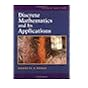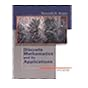Normal view

# Discrete mathematics and its applications / Kenneth H. Rosen.

Material type:TextLanguage: English Publication details: c2003. Edition: 5th edDescription: xxi, (various pagings) : ill. ; 26 cmISBN: 0072424346 (acidfree paper); 9780072424348; 0071198814Subject(s): Mathematics | Computer science -- MathematicsDDC classification: 511 LOC classification: QA39.3 | .R67 2003Online resources: WorldCat details | E-book Fulltext
Contents:
TOC The foundations: logic and proof, sets, and functions : Logic ; Propositional equivalences ; Predicates and quantifiers ; Nested quantifiers ; Methods of proof ; Sets ; Set operations ; Functions -- The fundamentals: algorithms, the integers, and matrices : Algorithms ; The growth of functions ; Complexity of algorithms ; The integers and division ; Applications of number theory ; Matrices -- Mathematical reasoning, induction, and recursion : Proof strategy ; Sequences and summations ; Mathematical induction ; Recursive definitions and structural induction ; Recursive algorithms ; Program correctness -- Counting : The basics of counting ; The pigeonhole principle ; Permutations and combinations ; Binomial coefficients ; Generalized permutations and combinations ; Generating permutations and combinations -- Discrete probability : An introduction to discrete probability ; Probability theory ; Expected value and variance -- Advanced counting techniques : Recurrence relations ; Solving recurrence relations ; Divide-and-conquer algorithms and recurrence relations ; Generating functions ; Inclusion-exclusion ; Applications of inclusion-exclusion -- Relations : Relations and their properties ; n-ary relations and their applications ; Representing relations ; Closures of relations ; Equivalence relations ; Partial orderings -- Graphs : Introduction to graphs ; Graph terminology ; Representing graphs and graph isomorphism ; Connectivity ; Euler and Hamilton paths ; Shortest-path problems ; Planar graphs ; Graph coloring -- Trees : introduction to trees ; Applications of trees ; Tree traversal ; Spanning trees ; Minimum spanning trees -- Boolean algebra : Boolean functions ; Representing Boolean functions ; Logic gates ; Minimization of circuits -- Modeling computation : Languages and grammars ; Finite-state machines with output ; Finite-state machines with no output ; Language recognition ; Turing machines -- Appendixes : A.1. Exponential and logarithmic functions ; A.2. Pseudocode.
Summary: This text] is appropriate for a one- or two-term introductory discrete mathematics course to be taken by students in a wide variety of majors, including computer science, mathematics, and engineering. College Algebra is the only explicit prerequisite.-Pref.
Tags from this library: No tags from this library for this title.
Star ratingsAverage rating: 0.0 (0 votes)
Holdings
Item type Current library Collection Call number Copy number Status Date due Barcode Item holdsE-Book
E-book
Non-fiction 511 ROD 2003 (Browse shelf(Opens below)) Not for loanText
Reserve Section
Non-fiction 511 ROD 2003 (Browse shelf(Opens below)) C-1 Not For Loan 10971Text
Reserve Section
Non-fiction 511 ROD 2003 (Browse shelf(Opens below)) C-2 Not For Loan 10972Text
Reserve Section
Non-fiction 511 ROD 2003 (Browse shelf(Opens below)) C-3 Not For Loan 10973Text
Circulation Section
Non-fiction 511 ROD 2003 (Browse shelf(Opens below)) C-4 Available 10974Text
Circulation Section
Non-fiction 511 ROD 2003 (Browse shelf(Opens below)) C-6 Checked out 18/04/2019 10976Text
Circulation Section
Non-fiction 511 ROD 2003 (Browse shelf(Opens below)) C-7 Available 10977Text
Circulation Section
Non-fiction 511 ROD 2003 (Browse shelf(Opens below)) C-8 Available 10978Text
Circulation Section
Non-fiction 511 ROD 2003 (Browse shelf(Opens below)) C-9 Available 10979Text
Circulation Section
Non-fiction 511 ROD 2003 (Browse shelf(Opens below)) C-10 Available 10980Text
Circulation Section
Non-fiction 511 ROD 2003 (Browse shelf(Opens below)) C-11 Available 10981Text
Circulation Section
Non-fiction 511 ROD 2003 (Browse shelf(Opens below)) C-12 Available 10982Text
Circulation Section
Non-fiction 511 ROD 2003 (Browse shelf(Opens below)) C-13 Available 10983Text
Circulation Section
Non-fiction 511 ROD 2003 (Browse shelf(Opens below)) C-14 Available 10984Text
Non-fiction 511 ROD (Browse shelf(Opens below)) C-15 Available 135-10985Text
Circulation Section
Non-fiction 511 ROD 2003 (Browse shelf(Opens below)) C-16 Available 10986Text
Circulation Section
Non-fiction 511 ROD 2003 (Browse shelf(Opens below)) C-18 Available 10988Text
Circulation Section
Non-fiction 511 ROD 2003 (Browse shelf(Opens below)) C-19 Available 10989
Total holds: 0
##### Browsing EWU Library shelves, Shelving location: Circulation Section Close shelf browser (Hides shelf browser)511 ROD 1999 Discrete mathematics and its applications / 511 ROD 2003 Discrete mathematics and its applications / 511 ROD 2003 Discrete mathematics and its applications / 511 ROD 2003 Discrete mathematics and its applications / 511 ROD 2003 Discrete mathematics and its applications / 511 ROD 2003 Discrete mathematics and its applications / 511 ROD 2003 Discrete mathematics and its applications /

Includes bibliographic references and indexes.

TOC The foundations: logic and proof, sets, and functions : Logic ; Propositional equivalences ; Predicates and quantifiers ; Nested quantifiers ; Methods of proof ; Sets ; Set operations ; Functions --
The fundamentals: algorithms, the integers, and matrices : Algorithms ; The growth of functions ; Complexity of algorithms ; The integers and division ; Applications of number theory ; Matrices --
Mathematical reasoning, induction, and recursion : Proof strategy ; Sequences and summations ; Mathematical induction ; Recursive definitions and structural induction ; Recursive algorithms ; Program correctness --
Counting : The basics of counting ; The pigeonhole principle ; Permutations and combinations ; Binomial coefficients ; Generalized permutations and combinations ; Generating permutations and combinations --
Discrete probability : An introduction to discrete probability ; Probability theory ; Expected value and variance --
Advanced counting techniques : Recurrence relations ; Solving recurrence relations ; Divide-and-conquer algorithms and recurrence relations ; Generating functions ; Inclusion-exclusion ; Applications of inclusion-exclusion --
Relations : Relations and their properties ; n-ary relations and their applications ; Representing relations ; Closures of relations ; Equivalence relations ; Partial orderings --
Graphs : Introduction to graphs ; Graph terminology ; Representing graphs and graph isomorphism ; Connectivity ; Euler and Hamilton paths ; Shortest-path problems ; Planar graphs ; Graph coloring --
Trees : introduction to trees ; Applications of trees ; Tree traversal ; Spanning trees ; Minimum spanning trees --
Boolean algebra : Boolean functions ; Representing Boolean functions ; Logic gates ; Minimization of circuits --
Modeling computation : Languages and grammars ; Finite-state machines with output ; Finite-state machines with no output ; Language recognition ; Turing machines --
Appendixes : A.1. Exponential and logarithmic functions ; A.2. Pseudocode.

This text] is appropriate for a one- or two-term introductory discrete mathematics course to be taken by students in a wide variety of majors, including computer science, mathematics, and engineering. College Algebra is the only explicit prerequisite.-Pref.

BA

Saifun Momota

There are no comments on this title.